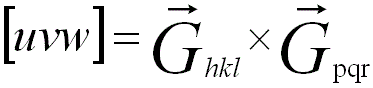Zone Axis Determined by Cross-product
- Practical Electron Microscopy and Database -
- An Online Book -
Microanalysis | EM Book                                                                                   https://www.globalsino.com/EM/

This book (Practical Electron Microscopy and Database) is a reference for TEM and SEM students, operators, engineers, technicians, managers, and researchers.
=================================================================================

In crystallographic analysis, a zone axis can be obtained by the cross-product of two vectors (or called faces), e.g. vectors (faces) [hkl] and [pqr] belong to zone [k·r-l·q, l·p-h·r, h·q-k·p]. In this case, the geometry of the zone axis [u v w] and the planes (h k l) and (p q r), which belong to the zone, is shown in Figure 2967. The line (their zone axis) of the intersection of the two planes is given by the cross product of their normals (reciprocal lattice vectors):--------------------- [2967a]
Then,
u = k·r - l·q --------------------- [2967b]
v = l·p - h·r --------------------- [2967c]
w = h·q - k·p --------------------- [2967d]

Here, the "x" in Equation 2967a denotes a cross product.Figure 2967. Zone axis [u v w] of the planes with indices (h k l) and (p q r).

Any other vector (face) [efg] belongs to the same zone if it is some linear combination of [hkl] and [pqr], for instance, e = 2h-3p, f = 2k-3q, etc.

For instance, vectors  and  belong to [1·0-0·1, 0·0-1·0, 1·1-1·0], or  (e.g. BCC). The zone axis of vectors  and  is [1·4-1·2, 1·1-2·4, 2·2-1·1] or [2,-7,1]. And, the vectors (faces) , , [1,-1,-3], etc. also belong to this zone.

A common cross-product formula to find a zone axis is given by:
[u v w] = [h k l] × [p q r] --------------------- [2967e]
Here, u, v and w are obtained in Equations 2967b - 2967d. Note that you can download the excel file for your own calculations.

For any plane (hkl) that belongs to the zone axis [u v w], the following relationship is satisfied:

hu + kv + lw = 0 --------------------- [2967f]

=================================================================================
The book author (Yougui Liao) welcomes your comments, suggestions, and corrections, please click here for submission. If you let book author know once you have cited this book, the brief information of your publication will appear on the “Times Cited” page.